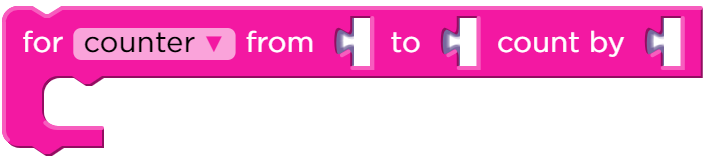Category:Loops

Repeats a sequence of code a specified number of times, and tracks a value each time the loop is run.

Sometimes we want to repeat things a certain number of times, but we want to keep track of values as we do. For loops are different than repeat loops because they use a counter variable to track a value. That value can then be used in the code inside the block.

The counter variable begins with the starting value. Each time the program goes through the loop, the sequence of code inside the block is run. The step value is then added to the counter variable, and the loop continues to run until the counter reaches the stopping value. Once the for loop is finished, the program will continue where it left off.

Examples:

for counter from 1 to 4 count by 1
The loop will run 4 times.

1. counter = 1

2. counter = 2

3. counter = 3

4. counter = 4

for counter from 3 to 9 count by 3
The loop will run 3 times.

1. counter = 3

2. counter = 6

3. counter = 9

## Parameters

Name Description
variable The name of the variable that keeps track of the number that controls the loop. Default name is “counter”
starting value The value the counter variable start at
ending value The value the counter variable must reach before the loop will end
step value The value to add to the counter variable each time the loop is run

## Tips

• The counter variable will be increased after the sequence of code is run, and the loop will stop when the value is equal to or greater than the stopping value. For example, if a counter starts at 3, ends at 6, and has an interval of 2, the loop will run twice - when the counter value is 3 and 5. The counter will end with a value of 7, but the loop will not run again because it is greater than the stopping value.

Found a bug in the documentation? Let us know at documentation@code.org